Many geometry problems either directly ask about the degree of certain angles or will require you to figure out the degree of an angle in the course of solving some problem. In successive posts, we will cover the basics of what an angle is, how we can use parallel lines to find unknown angles, and how we can use triangles/circles/polygons to find unknown angles.

Angles: Some Preliminaries

To understand angles, we need to begin with the idea of a line:

Line: a straight line that continues in both directions without end.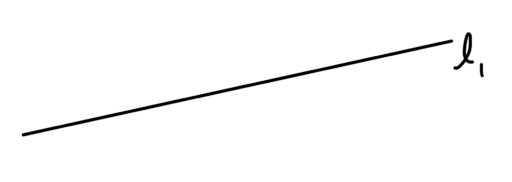Now, an angle appears where two lines intersect. More formally,

Angle: a measure of how much you would need to turn one line to make them part of the same line

For example,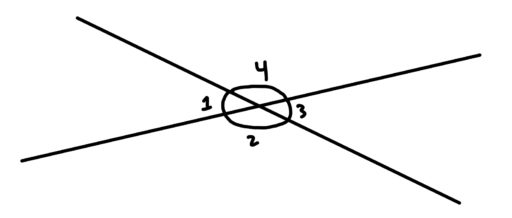And as you can see in the above diagram, there are actually four angles formed when two lines intersect. So there is a convention for naming each of the four angles: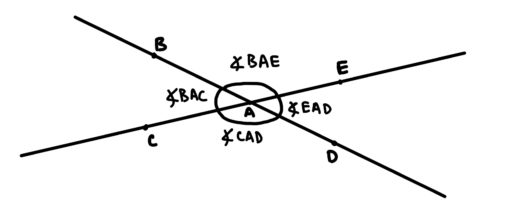[Practice problems with matching angles: label points on the lines and degree measures and have them match the two]

Now, here are two important facts about angles:

Lines Have 180 Degrees: The angle formed by a single line has 180 degrees.Circles Have 360 Degrees: The angle formed by a circle is 360 degrees.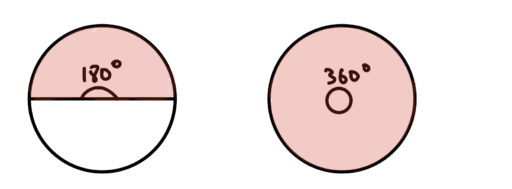Now, we also have two relationships among lines which are very important:

Parallel Lines: Parallel lines go in the same direction and therefore never intersect. We often denote that linesandare parallel by writing, as in the below diagram: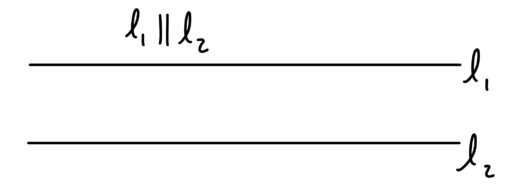Perpendicular Lines: Perpendicular lines intersect at a 90 degree angle. We denote two lines as perpendicular by writing: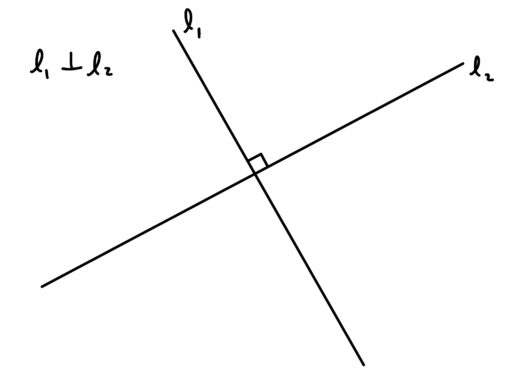In our next post, we will talk about some of the relationships that exist between angles and lines and shapes.